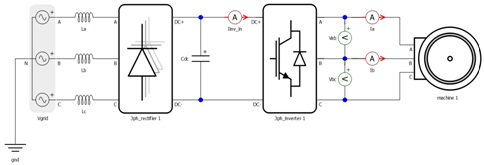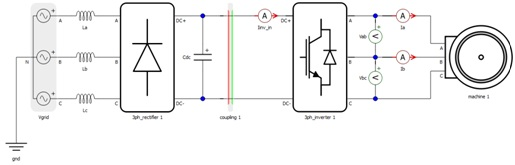# Irregular Model Example

This section describes irregular model example

Model depicted in Figure 1 will fail to compile due to too high overall weight of converters in a single sub- circuit. The issue can be fixed by dividing the circuit into two sub-circuits where each sub-circuit holds only one converter as depicted in Figure 2.Figure 1. Irregular example: the overall weight of converters in the sub-circuit is 6Figure 2. Fixed model: the overall weight of converters per sub-circuit is 3